Terminology:

• Light is a form of energy, which gives us the power of vision.
• Ray of light: It is a line in the direction of movement of light.
• The beam of light: It is the bunch of rays of light.
• Parallel beam: All the rays are parallel.

Reflection of Light

When the light is allowed to fall on the highly polished surface, such as a mirror, most of the light gets reflected.

Laws of Reflection

• 1. The angle of incidence is always equal to the angle of reflection.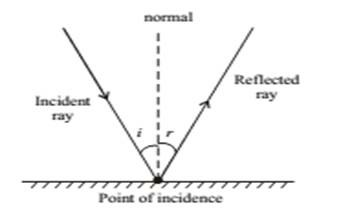• The incident ray reflected ray and the normal to the reflecting surface at the point of incidence lie in the same plane.

The image formed by Plane Mirror (Plane reflecting surface)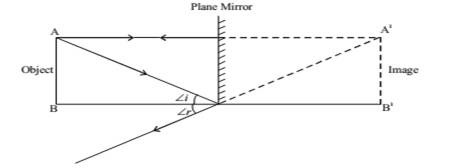• Virtual (imaginary) & Erect: The image that does not form on the screen. Real images can be recorded on the screen.
• Laterally inverted (The left side of the object appear on right side of image)
• The size of the image is equal to that of the object.
• The image formed is as far behind the mirror as the object is in front of it.

Reflection of light by Spherical Mirrors

Mirrors, whose reflecting surface are curved inward or outward spherically are called a spherical mirror. For example – Spoon. The curved surface of the shinning spoon can be considered as a curved mirror.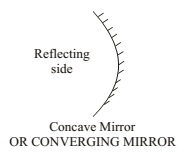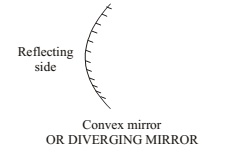If it is curved inward -> Act as a concave mirror

If it is curved outward -> Act as a convex mirror.

Few Basic terms related to Spherical Mirror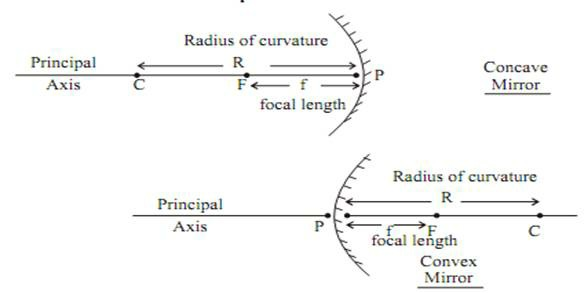• Principal axis: Line joining the pole and centre of curvature of the spherical mirror.
• Pole: The geometrical central point of the reflecting spherical surface. (aperture), denoted by (P).
• Aperture: The width of the reflecting spherical surface.
• Centre of curvature: The centre of the hollow glass sphere of which the spherical mirror is a part is called the centre of curvature.
• The radius of curvature: The distance between the pole and the centre of curvature. i.e. PC = R or The radius of the hollow sphere of which the mirror is a part.
• Focus point: The point on the principal axis, where all parallel rays meet after reflection is called as Principal Focus or Focus. It is denoted by letter ‘F’.
• Focal length: The distance between the pole and focus point
i.e. PF = f

Image Formation by Spherical Mirror

Before we learn the formation of image or ray diagram, let us go through few tips

• Remember, a ray of light which is parallel to principle axis always pass through focus (meet at focus) or vice-versa.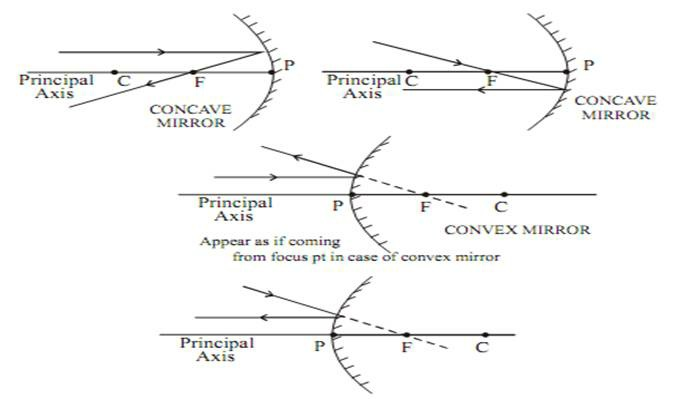• A ray of light which passes through the centre of curvature (it is also known as normal at the point of incidence on a spherical mirror) will retrace their path after reflection.
• A ray of light falling on pole get reflected at the same angle on the other side of the principal axis.

Note: A ray of light passes through the centre of curvature of reflecting spherical surface always act as normal at the point of incidence. If we know the normal we can draw an angle of incidence and angle of reflection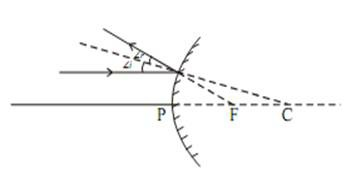Note: The image will only form when two or more rays meet at a point. Image formation by a concave mirror for the different position of the object.

The ray diagrams given in NCERT Books are also good to be followed.

Image Formation by Concave Mirror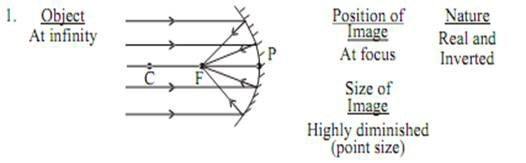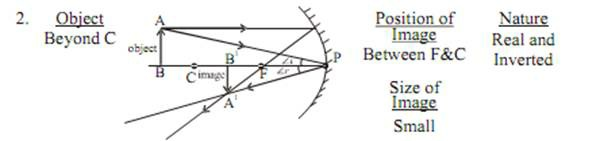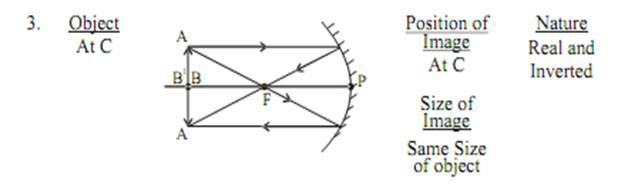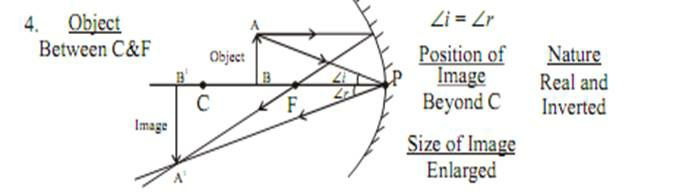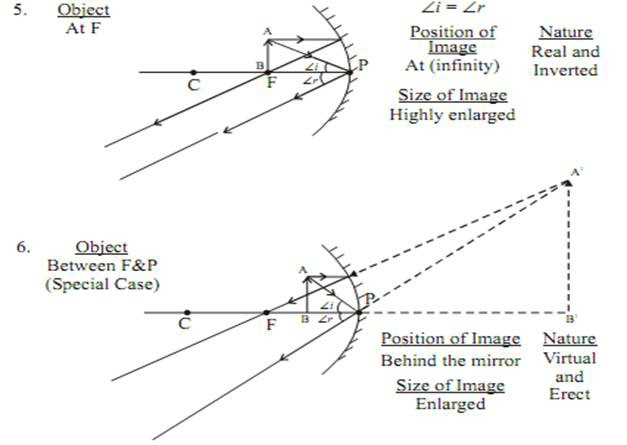Image formation by Convex Mirror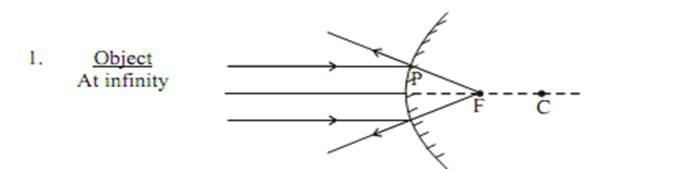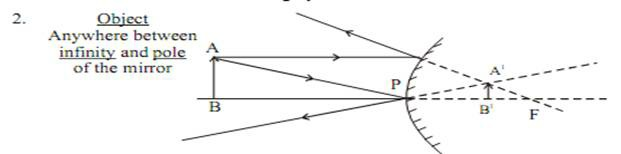Uses of Concave Mirror

• Used in torches, searchlight and headlight of the vehicle.
• Used to see the large image of a face as a shaving mirror
• Used by the dentist to see large images of the teeth
• The large concave mirror used to focus sunlight (heat) in solar furnaces.

Uses of Convex Mirror

Used as a rear-view mirror in vehicles because it gives an erect image. It also helps the driver to view a large area.

Sign Convention for Reflection by Spherical Mirror

• The object is always placed to the left side of the mirror.
• All distance should be measured from the pole (P); parallel to the principal axis.
• Take ‘P’ as the origin. Distances measured.
• The right of the origin (+x-Axis) is taken positively.
• Left of the origin (–x-Axis) are taken negatively.

Perpendicular to and above principal axis (+y-Axis) are taken positive Perpendicular to and below principal axis (–y-Axis) are taken negative

Mirror Formula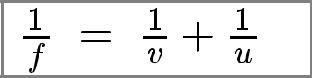f -> distance between F and Pole

v -> distance of image from Pole

u -> distance of the object from Pole

R -> distance between the centre of curvature and pole.

Magnification

It is expressed as the ratio of the height of the image to height of the object.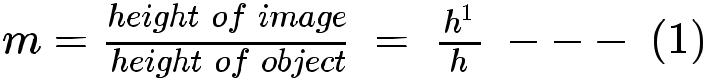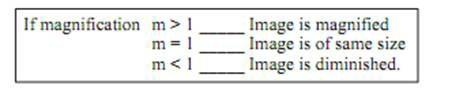h – is always +ve

h´ – is +ve for virtual, –ve for Real.